Next: BCS Theory Up: Superconductivity Previous: Superconductivity

# London Theory

The London equations  deal with the electrodynamic behaviour of superconductors on a macroscopic scale. They relate the local electric ( E) and magnetic ( B) fields to the supercurrent density  J as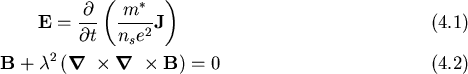The supercurrent density  J is due to the number density ns of carriers (either electrons or holes, depending on the superconductor) of effective mass m* which encounter no resistance. The London penetration depth, defined as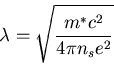(4.1)

is the only free parameter in these equations. The first London equation (2.1) expresses perfect conductivity in that the electric field accelerates the superelectrons. The second equation describes the Meissner effect. It implies that within a superconductor an external magnetic field decays over the characteristic distance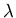. An example of this is the situation illustrated in Figure 2.1, where a constant applied field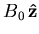exists parallel to the surface at x = 0 of a superconductor.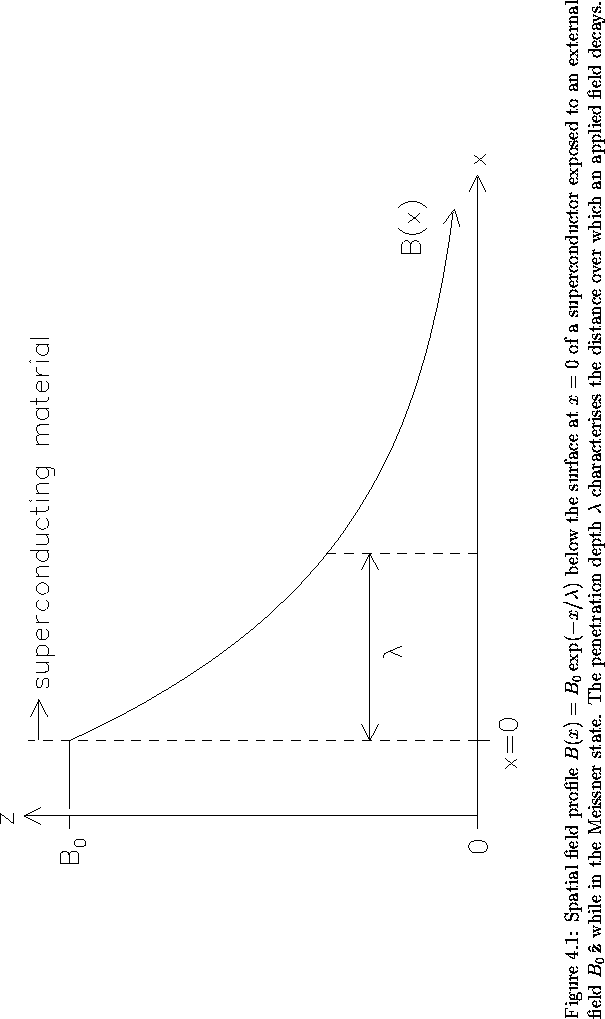With the aid of the vector identity

 (4.2)

and one of Maxwell's equations,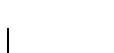, equation (2.2) becomes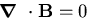(4.3)

Inside the superconductor the solution for the internal field B is then, as plotted in Figure 2.1. With slight modification these London equations continue to be valid in the presence of magnetic vortices.

The spatial magnetic field profile of a vortex lattice can be modelled with a generalized London theory. For an array of flux lines at positions ri, expression (2.2) becomes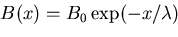(4.4)

This equation portrays each filament of one flux quantum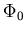as having the proportions of a two-dimensional delta function, leading to unrealistic field and supercurrent divergences at the vortex centre. Such unphysical behaviour disappears through the incorporation of finite core size, often achieved by multiplying Fourier components of the field B with a cutoff factor in reciprocal space. Equation (2.6) applies when the external field H lies far enough from the upper critical field Hc2that the vortices are substantially more than the radius of a physical vortex apart. The internal field then consists of the superposition of the contributions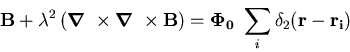(4.5)

from each flux line i, whereis the Hankel function of imaginary argument of zero order. An explanation of the flux line lattice geometry transitions induced by external field changes in superconductors like LuNi2B2C demands a further extension of the London equations .

Nonlocal electrodynamics accounts for the dependence of the supercurrent density J(r) on the vector potential A(r) within a characteristic range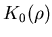from the point  r . The effects of nonlocality are significant in regions where the vector potential A(r)varies rapidly within a volume of radius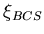, provided that the temperature is low and the mean free path l exceeds this radius. Otherwise scattering suppresses nonlocal effects . The basic London equations (2.1) and (2.2) derive from the local relation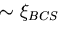(4.6)

by taking the time derivative and the curl respectively  or, in terms of spatial Fourier components ,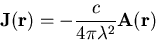(4.7)

Inclusion of nonlocality transforms this equation to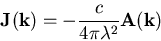(4.8)

where the expression for the kernel Q(k) emerges from the microscopic approach of the Bardeen-Cooper-Schrieffer (BCS) theory.Next: BCS Theory Up: Superconductivity Previous: Superconductivity
Jess H. Brewer
2001-10-31